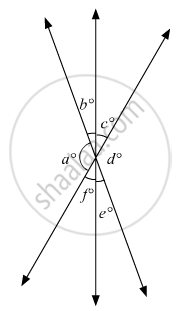# In the Given Figure, Which of the Following Statements Must Be True? (I) a + B = D + C (Ii) a + C + E = 180° (Iii) B + F = C + E - Mathematics

MCQ

In the given figure, which of the following statements must be true?
(i) a + b = d + c
(ii) a + c + e = 180°
(iii) b + f = c + e#### Options

•  (i) only

•  (ii) only

•  (iii) only

• (ii) and (iii) only

#### Solution

Now, let us consider each statement one by one:(i) Statement:  a+b+ = d+ c

This statement is incorrect

Explanation:

We have, a and d are vertically opposite angles.

Therefore,

a = d (I)

Similarly, b and e are vertically opposite angles.

Therefore,

b =e (II)

On adding (I) and (II), we get:

a + b = d + e

Thus, this statement is incorrect.

(ii) Statement:  a+ c+ e = 180°

This statement is correct.

Explanation:

As  a°, f° and e°form a linear pair, therefore their sum must be supplementary.

a +f +e = 180° (III)

Also f°and c°are vertically opposite angles, therefore, these must be equal.

f = c

Putting f = c in (III), we get:

a + c + e = 180°

(iii) Statement:  b + f = a + e

This statement is correct.’

Explanation:

As a°, f° and b° form a linear pair, therefore their sum must be supplementary.

a + f + b = 180°   (IV)

Also c°, d° and e° form a linear pair, therefore their sum must be supplementary.

c + d + e = 180° (V)

On comparing (IV) and (V), we get:

a+ f +b = c + d + e

Also  a°and d°are vertically opposite angles, therefore, these must be equal.

Therefore,

a = b

Substituting the above equation in (VI), we get:

a + f + b = c + d + e

a + f + b = c+ a + e

b + f = a + e

Thus, out of all, (ii) and (iii) are correct.

Concept: Concept of Parallel Lines
Is there an error in this question or solution?

#### APPEARS IN

RD Sharma Mathematics for Class 9
Chapter 10 Lines and Angles
Q 9 | Page 52